#### Theory of Ebers-Moll BJT model and its Modes of Operation

Introduction:

The bipolar junction transistor can be considered basically as two p-n junctions placed back-to-back, with base p-type region being general to both diodes. This can be seen as two diodes containing a common third terminal as shown in figure below.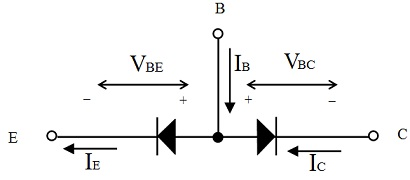Figure: Bipolar Transistor Shown as Two Back-to-Back p-n Junctions

Though, the two diodes are not in isolation, however are interdependent. This signifies that the total current flowing in each and every diode is influenced by the conditions prevailing the other. In isolation, the two junctions would be characterized by normal Diode Equation with an appropriate notation employed to differentiate among the two junctions as can be seen in figure below. If the two junctions are joined, though, to form a transistor, the base region is shared internally by both diodes even although there is an external connection to it. As seen formerly, in forward active mode, αF of emitter current reaches the collector. This signifies that αF of the diode current passing via the base-emitter junction contributes to current flowing via the base-collector junction. Usually, αF has a value between 0.98 and 0.99. This is as shown as forward component of current as it applies to normal forward active mode of the operation of device. Note that this current is shown as a conventional current in figure below. This is equally possible to reverse the biases on junctions to operate the transistor in “reverse active mode”. In this situation, αR times the collector current will contribute to emitter current. For doping, ratios generally used the transistor will be much less efficient in the reverse mode and αR would usually be in the range of 0.1 to 0.5.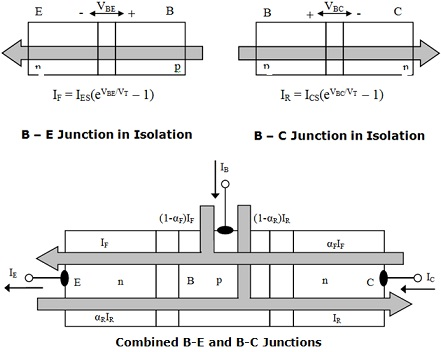Figure: The n-p-n Transistor Considered as combined p-n Junctions

Ebers-Moll Equations:

The Ebers-Moll transistor model is an effort to form an electrical model of the device as two diodes whose currents are recognized by the normal diode law however with additional transfer ratios to quantify the interdependency of the junctions as shown in figure below. Two dependent current sources are employed to point out the interaction of junctions. The interdependency is quantified by the forward and reverse transfer ratios, αF and αR. The diode currents are as follows:

IF = IES (eVSE/VT - 1) where IES = qA [(Depeo/Le) + (Dbnbo/Wb)] = qA [(Deni2/LeNe) + (Dbni2/WbNb)]

IR = ICS (eVBC/VT - 1) where ICS = qA [(Dcpco/Lc) + (Dbnbo/Wb)] = qA [(Dcni2/LcNc) + (Dbni2/WbNb)]

By applying the Kirchoff’s laws to the model gives the terminal currents as shown:

IE = IF - αRIR        αF = 0.98 – 0.99 typically
IC = αFIF – IR        αR = 0.1 – 0.5 typically
IB = IE - IC

This gives,

IE = IES (EVBE/VT - 1) – αR ICS (EVBC/VT - 1)

IC = αF IES (EVBE/VT - 1) – ICS (EVBC/VT - 1)

IB = (1 - αF) IES (EVBE/VT - 1) + (1 - αR) ICS (EVBC/VT - 1)

These are termed as the Ebers-Moll Equations for bipolar transistor (shown in figure below).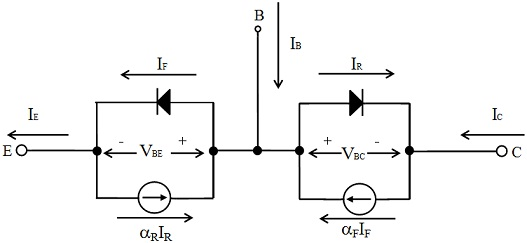Figure: The Ebers-Moll Model of an n-p-n Bipolar Junction Transistor

IE = IES (EVBE/VT - 1) – αR ICS (EVBC/VT - 1)

IC = αF IES (EVBE/VT - 1) – ICS (EVBC/VT - 1)

IB = (1 - αF) IES (EVBE/VT - 1) + (1 - αR) ICS (EVBC/VT - 1)

Modes of Operation:

The Ebers-Moll BJT Model is an excellent big-signal, steady-state model of the transistor and permits the state of conduction of device to be simply determined for various modes of operation of the device. The various modes of operation are recognized by the way in which the junctions are biased. The charge profiles for each and every mode are shown in figure below.

(a) Forward Active Mode:

B-E forward-biased and VBE positive             B-C reverse biased and VBC negative

eVBE/VT >> 1                                                  eVBC/VT << 1

Then from the model, we get

IE ≈ IES eVBE/VT     Relatively large

IC ≈ αF IES eVBE/VT = αF IE    Relatively large

IB ≈ (1 - αF) IES eVBE/VT = (1 - α) IE   Small

(b) Reverse Active Mode:

B-E reverse biased and VBE negative       B-C forward biased and VBC positive

eVBE/VT << 1,                                           eVBC/VT >> 1

Basically the transistor conducts in opposite direction.

From the model,

IE ≈ - αR ICS eVBC/VT     Moderately high

IC ≈ - ICS eVBE/VT     Moderate

IB ≈ (1 – αR) ICS eVBC/VT   As big as 0.5 |IC|

This mode doesn’t give useful amplification however is used, mainly, for the current steering in switching circuits, example: TTL.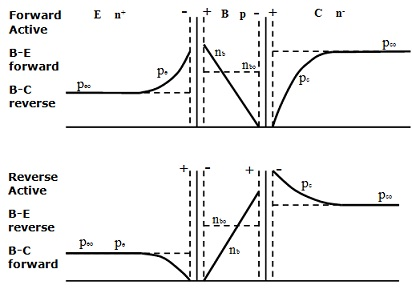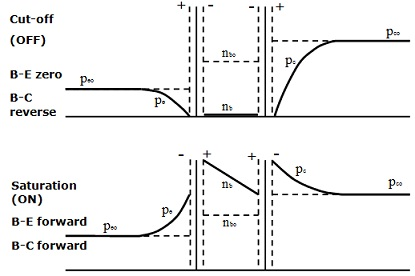Figure: Charge Profiles for Modes of Operation of n-p-n BJT

(c) Cut-Off Mode:

B-E unbiased and VBE = 0V              B-C reverse biased and VBC negative

eVBE/VT = 1,                                     eVBC/VT << 1.

Then,

IE ≈ αR ICS   Leakage current nA

IC ≈ ICS   Leakage current nA

IB ≈ - (1 - αR) ICS

This is equal to a very low conductance among collector and emitter as shown in figure below, that is, an open switch.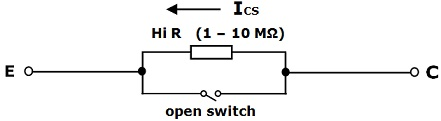Figure: The Cut-Off Mode of Operation as equal to a Leaky Switch

(d) The Saturation Mode:

B-E forward biased and VBE positive            B-C forward biased and VBC positive

eVBE/VT >> 1                                                eVBC/VT >> 1

Note: If both the junctions are forward biased then,

IE ≈ IES eVBE/VT - αR ICS eVBC/VT

IC ≈ αF IES eVBE/VT - ICS eVBC/VT

IB ≈ (1 - αF) IES eVBE/VT + (1 - αR) ICS eVBC/VT

In this situation, with both junctions forward biased:

VBE ≈ 0.8 V
VBC ≈ 0.7 V
VCE = VBE – VBC = 0.1 V

There is a 0.1V drop across transistor from collector to emitter that is much low whereas a substantial current flows via the device. In this mode, it can be believed as containing a very high conductivity and acts as a closed switch with finite conductivity or resistance.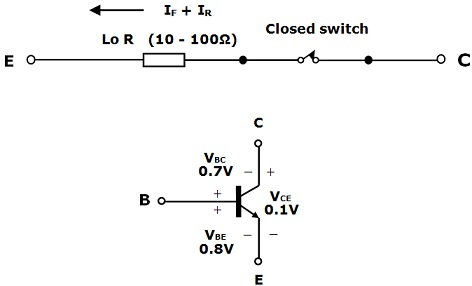Figure: Saturation Mode of Operation Equivalent to a Closed Switch

Latest technology based Electrical Engineering Online Tutoring Assistance

Tutors, at the www.tutorsglobe.com, take pledge to provide full satisfaction and assurance in Electrical Engineering help via online tutoring. Students are getting 100% satisfaction by online tutors across the globe. Here you can get homework help for Electrical Engineering, project ideas and tutorials. We provide email based Electrical Engineering help. You can join us to ask queries 24x7 with live, experienced and qualified online tutors specialized in Electrical Engineering. Through Online Tutoring, you would be able to complete your homework or assignments at your home. Tutors at the TutorsGlobe are committed to provide the best quality online tutoring assistance for Electrical Engineering Homework help and assignment help services. They use their experience, as they have solved thousands of the Electrical Engineering assignments, which may help you to solve your complex issues of Electrical Engineering. TutorsGlobe assure for the best quality compliance to your homework. Compromise with quality is not in our dictionary. If we feel that we are not able to provide the homework help as per the deadline or given instruction by the student, we refund the money of the student without any delay.